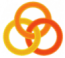General
 Course Home Page ESSLLI 2004Material
Lecture 1
 Slides Practical Session
Lecture 2
 Slides Practical Session
Lecture 3
 Slides Practical Session
Lecture 4
 Slides Practical Session
Lecture 5
 Slides Practical Session

# Hint

The predicate `concatenate/3` that we defined in the lecture or the built-in predicate `append/3`. (They do the same thing.)

In the lecture, we saw that `append` can be used to concatenate two given lists.

```?- append([a,b,c],[y,z],Out).
Out = [a,b,c,y,z]
```
But append can also be used in other ways. For example, to split lists into two parts.
```?- append(X,Y,[a,b,c]).
X = []
Y = [a,b,c] ;

X = [a]
Y = [b,c] ;

X = [a,b]
Y = [c] ;

X = [a,b,c]
Y = [] ;

no
```

How can this be used to define `prefix/2`?